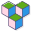CppBuzz# Java program to multiply two float numbers

``````public class Main {

public static void main(String[] args) {
float fNumber1 = 2.43f;
float fNumber2 = 3.68f;

System.out.println("Multiplication of two floating point numbers is : " + (fNumber1 * fNumber2));
}
}``````